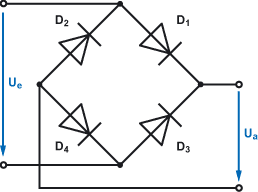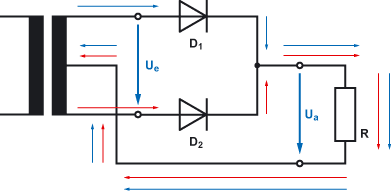# Rectifier circuitTo generate a DC voltage such as primary cells (batteries) and a secondary cell (battery). They produce a DC voltage by converting chemical energy into electrical energy.

The alternative is to generate a DC voltage of the AC voltage. This valve is made of the effect of the pn junction of a semiconductor diode.

Note: The following circuit is a resistor in series to the diode. Resistance is needed to perform measurements with an oscilloscope. Without resistance there will be no closed circuit.

Half-wave rectifier circuitHalf wave rectifier circuit. It consists of a simple diode. The polarity of the diode determines whether positive or negative voltage is applied to the output of the circuit.
The fact that the semiconductor diode, passing current in only one direction, locking the second half coming from the AC waveform.On receipt of the half wave rectifier circuit an ordinary sinusoidal AC voltage is applied.At the output of a half wave rectifier circuit produces a DC voltage pulse. Because current flows in one direction through the diode, which is missing in every second half cycle of alternating current in the output voltage U.
Under resistive load (resistance R) breaks pulsed DC voltage U DC with ripple.

Center two-way rectifier circuitThe center two-way rectifier circuit is called a two-pulse midpoint circuit M2. It requires a transformer with a center, in the current can flow back. The two diodes, the current of two half-cycles of the input voltage U e of a point out of the circuit. On a common line to the transformer, the currents are reduced.The current flow through the diode D 1 is unchanged on the Resistance. Because of the diode D 2 , it can not flow into the transformer.
The current flow through the second half wave is determined by the diode D 2 out. Through the diode D 1 , it can not flow to the transformer. The flow of the second half cycle is conducted through the resistor to the center tap.

Bridge rectifier circuitThe bridge rectifier circuit is also known as two-pulse bridge rectifier circuit B2. It consists of two parallel pairs of diodes. The AC input is located between the diode pairs.
The arrangement of the semiconductor diodes in the circuit of AC current flows in two different paths through the circuit.
The consumer is always traversed in one direction of flow.Through the diode circuit, the current flow through the second half wave is altered so that the output voltage U a pulsates. It is also known as pulsating DC voltage at which the second half cycle of input voltage U e is flipped up.
Under resistive load breaks the pulsating DC voltage U DC with a ripple.

Power supply is an electronic device that supplies electric energy to an electrical load. The primary function of a power supply is to convert one form of electrical energy to another and, as a result, power supplies are sometimes referred to as electric power converters. Some power supplies are discrete, stand-alone devices, whereas others are built into larger devices along with their loads. Examples of the latter include power supplies found in desktop computers and consumer electronics devices.